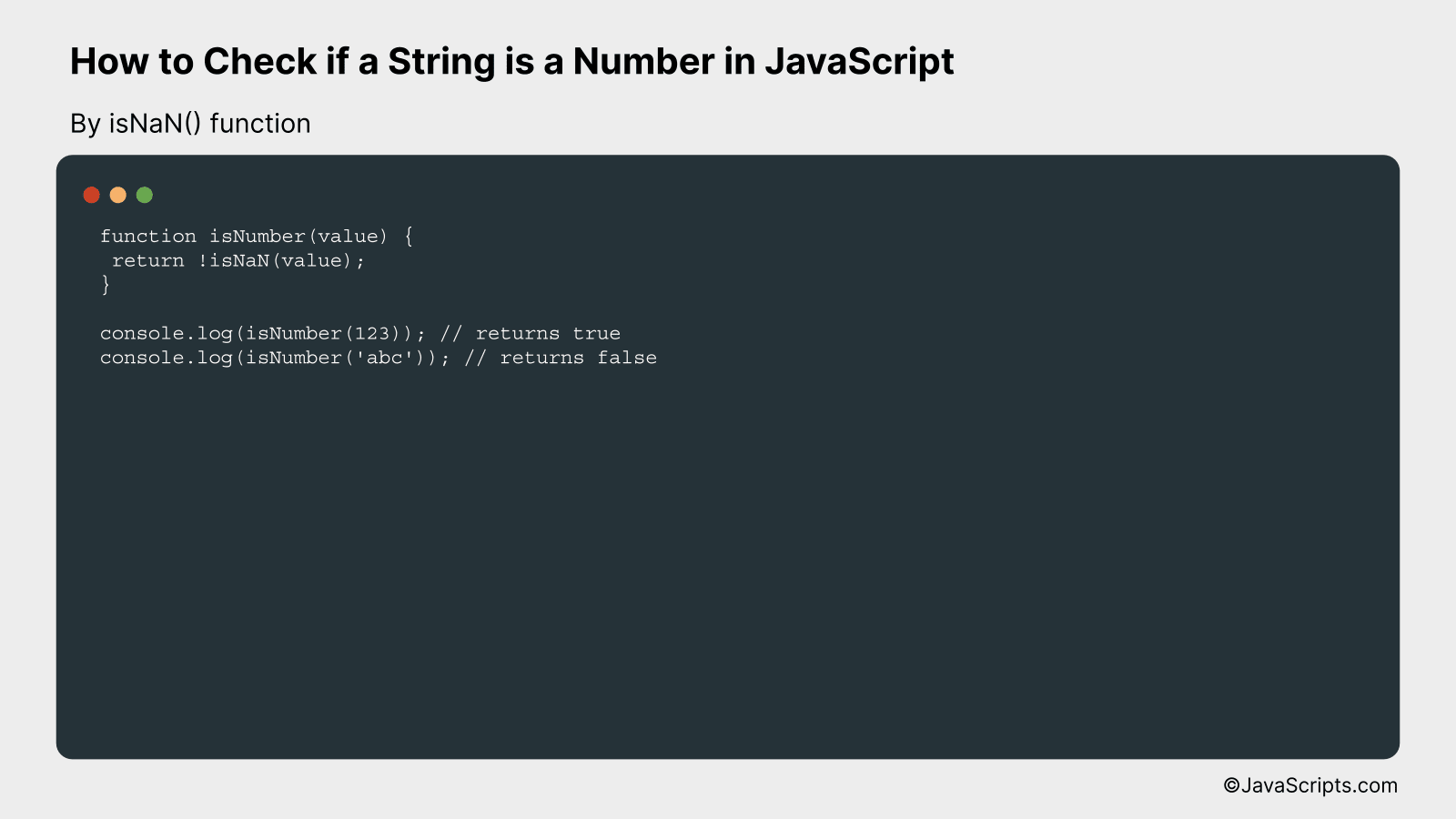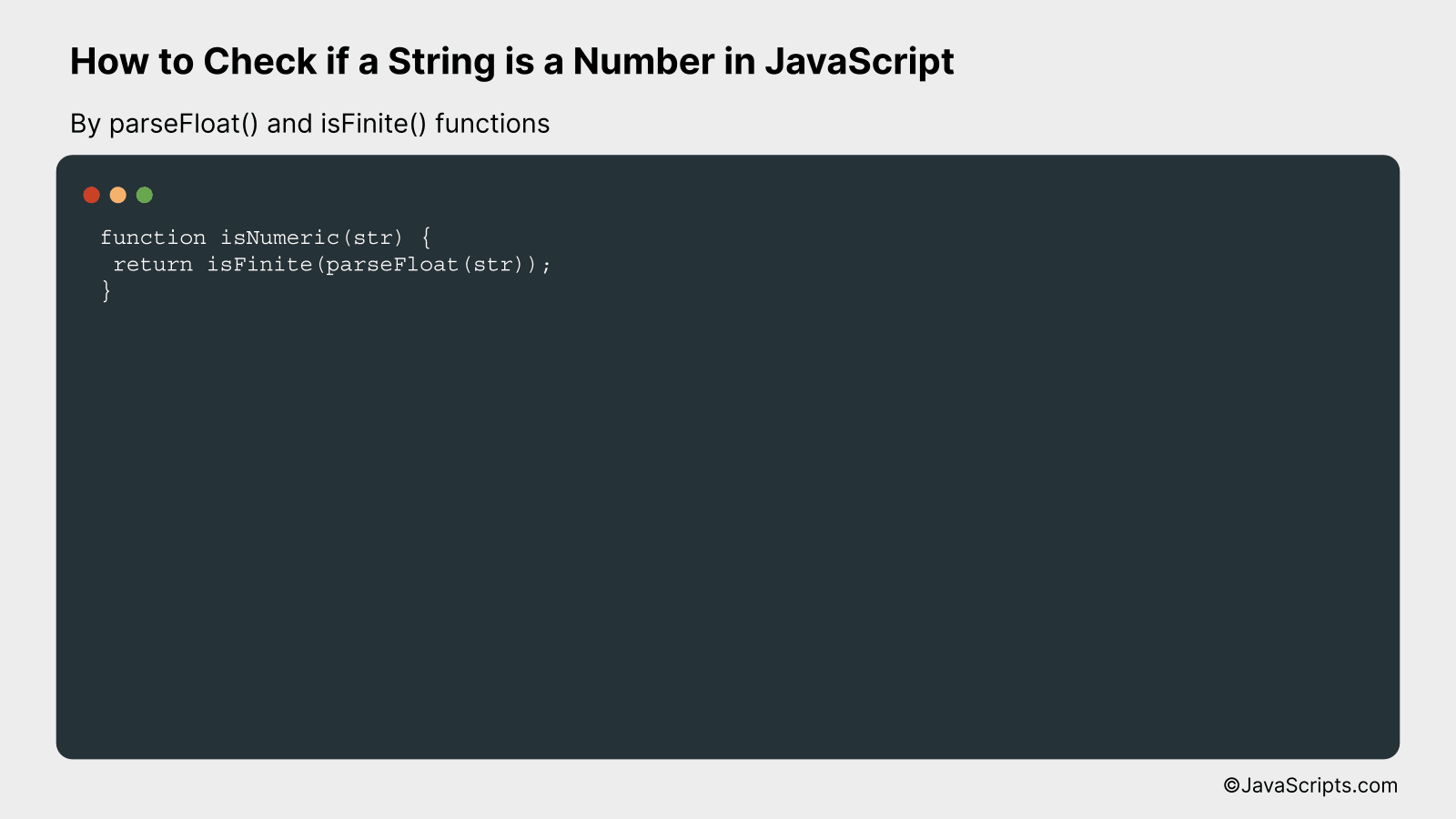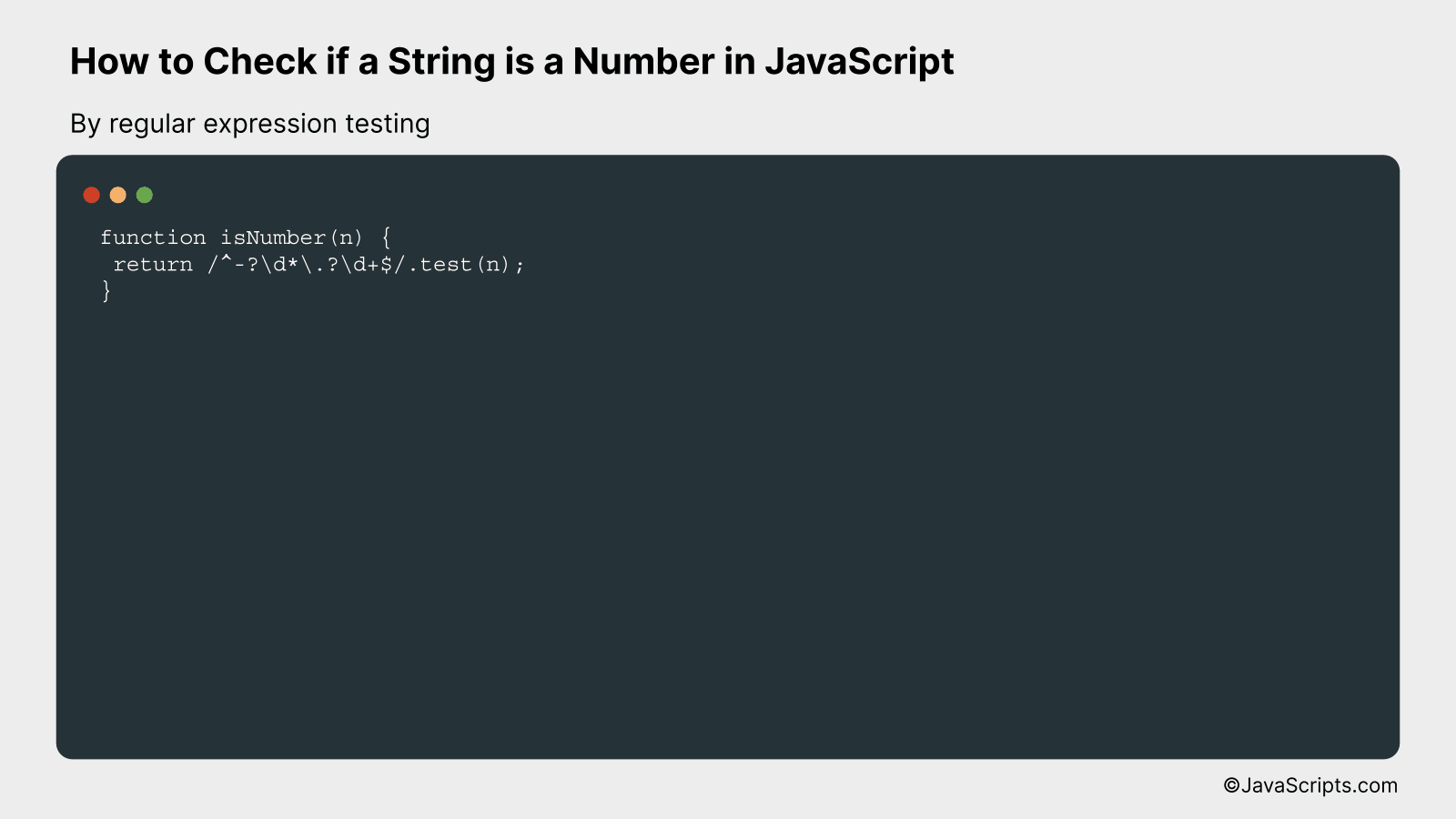# How to Check if a String is a Number in JavaScript

Ever found yourself scratching your head, pondering on how to decipher whether a string holds a numerical value or not in JavaScript? You’re not alone. Many budding developers like us encounter this situation while working on various coding projects.

Don’t worry, the solution is not as complex as it seems. We’ll walk through some straightforward techniques to check whether a string is a number or not in JavaScript. Stick around and let’s unravel this mystery together.

In this post, we will be looking at the following 3 ways to check if a string is a number in JavaScript:

• By isNaN() function
• By parseFloat() and isFinite() functions
• By regular expression testing

Let’s explore each…

## #1 – By isNaN() functionThe JavaScript isNaN() function can be used to check if a string is a number. This function returns true if the argument passed is not a number, and false if it is a number. For example, isNaN(123) returns false, whereas isNaN(‘abc’) returns true.

``````
function isNumber(value) {
return !isNaN(value);
}

console.log(isNumber(123)); // returns true
console.log(isNumber('abc')); // returns false
```
```

### How it works

The JavaScript isNaN() function checks whether a value is an illegal number (Not-a-Number). It returns true if the value is NaN, and false if it’s not. In our function, we use the negation operator (!) to reverse this, returning true when the value is a number, and false when it’s not.

• First, we define a function ‘isNumber’ that takes a single argument ‘value’.
• Inside the function, we return the opposite of the result of the isNaN() function on ‘value’. This is because isNaN() returns true for values that are NOT numbers, but we want our function to return true for numbers.
• When we call the function with a number, like ‘123’, isNaN(123) returns false (because 123 is a number), and !false gives us true. Hence, isNumber(123) returns true.
• When we call the function with a string that is not a number, like ‘abc’, isNaN(‘abc’) returns true (because ‘abc’ is not a number), and !true gives us false. Hence, isNumber(‘abc’) returns false.

## #2 – By parseFloat() and isFinite() functionsThe JavaScript code will determine whether a given string is a number, by first converting the string to a floating-point number using the parseFloat() function, and then checking if the result is a finite number using the isFinite() function. If the value is a valid number, it will be finite, but if the string contains non-numeric characters, the result will be NaN (Not a Number) and hence not finite. Here’s an example:

``````
function isNumeric(str) {
return isFinite(parseFloat(str));
}
```
```

### How it works

The isNumeric function works by first trying to convert the input string to a floating-point number using the JavaScript parseFloat() function. If the string can be successfully converted to a number, the output is that number. If it cannot be converted (because it contains non-numeric characters, for example), the output is NaN. The isFinite() function then checks whether this output is a finite number. Only valid numbers are finite, so the isFinite() function will return true if the string is a number, and false otherwise.

• Step 1: The input string is passed to the isNumeric function.
• Step 2: Inside the function, parseFloat() is called on the input string. This attempts to convert the string to a floating-point number.
• Step 3: If the string can be converted to a number, parseFloat() will return that number. If it cannot be converted, parseFloat() will return NaN.
• Step 4: isFinite() is then called on the result of parseFloat(). If the result is a finite number (i.e., any number except Infinity, -Infinity, and NaN), isFinite() will return true. If the result is not a finite number, isFinite() will return false.
• Step 5: The result of isFinite() is then returned by the isNumeric function. This will be true if the string could be converted to a finite number, and false otherwise.

## #3 – By regular expression testingThis method will employ JavaScript’s built-in function test() on a regular expression object to check if a string can be classified as a number. For instance, for the string ‘1234’, the function will return true, but for the string ‘1234a’, it will return false because it contains a non-numeric character.

``````
function isNumber(n) {
return /^-?d*.?d+\$/.test(n);
}
``````

### How it works

The function isNumber(n) takes a string as an argument and applies the test() function on it which is built into the JavaScript RegExp object. The test() function checks if the string matches the specified pattern, described by the regular expression ^-?d*.?d+\$, and returns true if it matches and false if it doesn’t.

• The ^ symbol at the beginning of the regular expression specifies that the match must start at the beginning of the string.
• The -? allows for an optional negative sign at the start.
• The d* allows for any number (including zero) of digits before a decimal point.
• The .? allows for an optional decimal point.
• The d+ requires one or more digits after the decimal point.
• The \$ symbol at the end of the regular expression specifies that the match must end at the end of the string.

This regular expression will match any string that represents a positive or negative integer or decimal number, and has no characters other than digits, a decimal point, or a negative sign.

Related:

In conclusion, understanding how to verify if a string is a number in JavaScript is an essential skill for developers. It not only helps to prevent errors but also optimizes the user experience by validating input data.

There are multiple methods to perform this check, such as by using the isNaN(), Number(), parseFloat(), and regular expressions. Remember, each technique may suit different situations. To choose the right one, understand the context and requirements of your code.